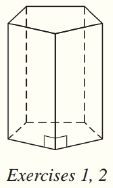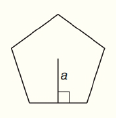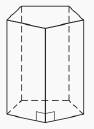# For the regular pentagonal prism shown below, find the total number of a) edges. ______ b) faces. _______### Elementary Geometry for College St...

6th Edition
Daniel C. Alexander + 1 other
Publisher: Cengage Learning
ISBN: 9781285195698### Elementary Geometry for College St...

6th Edition
Daniel C. Alexander + 1 other
Publisher: Cengage Learning
ISBN: 9781285195698

#### Solutions

Chapter
Section
Chapter 9.CT, Problem 1CT
Textbook Problem

## For the regular pentagonal prism shown below, find the total number of a) edges. ______ b) faces. _______Expert Solution
To determine

a)

To find:

The total number of edges for the regular pentagon prism.

Solution:

The total number of edges in the regular pentagon prism is 15.

### Explanation of Solution

Given:

The regular pentagon prism is shown below.’Calculation:

We see that the base of the regular pentagon prism is a regular pentagon.

We know that there are five edges in a regular pentagon.

Therefore one base of the regular pentagon prism has five edges.

Since there are two bases, there are 10 base edges.

Also, there are five lateral edges.

Thus the number of edges in the regular pentagon prism is 10+5=15 edges.

Final statement:

Therefore the total number of edges in the regular pentagon prism is 15.

Expert Solution
To determine

b)

To find:

The total number of faces for the regular pentagon prism.

Solution:

The total number of faces in the regular pentagon prism is 7.

### Explanation of Solution

Given:

The regular pentagon prism is shown below.’Calculation:

We see that there are two base faces and five lateral faces.

Hence the total number of faces is 7.

Final statement:

Therefore the total number of faces in the regular pentagon prism is 7.

### Want to see more full solutions like this?

Subscribe now to access step-by-step solutions to millions of textbook problems written by subject matter experts!

Get Solutions

### Want to see more full solutions like this?

Subscribe now to access step-by-step solutions to millions of textbook problems written by subject matter experts!

Get Solutions